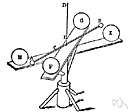universal gravitational constant

Also found in: Thesaurus, Medical, Acronyms, Encyclopedia, Wikipedia.
ThesaurusAntonymsRelated WordsSynonymsLegend:
 Noun 1universal gravitational constant - (physics) the universal constant relating force to mass and distance in Newton's law of gravitationlaw of gravitation, Newton's law of gravitation - (physics) the law that states any two bodies attract each other with a force that is directly proportional to the product of their masses and inversely proportional to the square of the distance between themnatural philosophy, physics - the science of matter and energy and their interactions; "his favorite subject was physics"constant - a number representing a quantity assumed to have a fixed value in a specified mathematical context; "the velocity of light is a constant"
References in periodicals archive ?
The Australians who did the original work, Frank Stacey of the University of Queensland in Brisbane and his collaborators, had found that in the mines, Newton's universal gravitational constant appeared slightly larger than the value determined by centuries of measurement in laboratories.
where T is the stress energy tensor, G is the Einstein curvature tensor and G is the universal gravitational constant. He notes the very large value of the proportionality constant.
where [[PHI].sub.e] = -GM/r is the gravitational scalar potential in a spherically symmetric gravitational field, r > R, the radius of the homogeneous sphere, G is the universal gravitational constant, c is the speed of light in vacuum, [m.sub.p] is the passive mass of the test particle, M is the mass of the static homogeneous spherical mass, [m.sub.0] is the rest mass of the test particle and u is the instantaneous velocity of the test particle.

Site: Follow: Share:
Open / Close